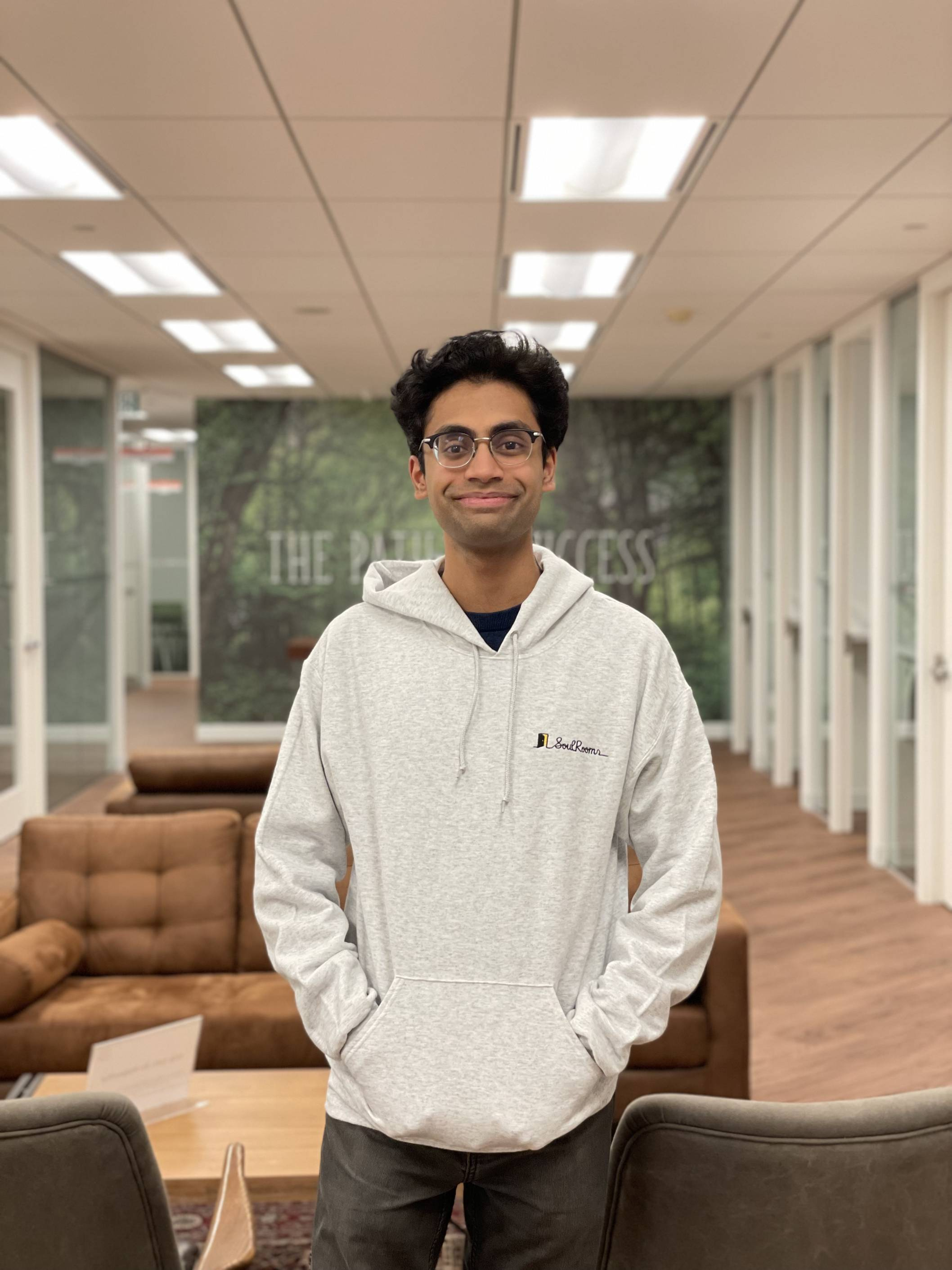Adithya ChakravarthyAboutResearchMath Videos

# Research

My research interests include Iwasawa theory, Galois representations, and $p$-adic $L$-functions. For my senior thesis, I studied growth patterns of Selmer groups and fine Selmer groups in infinite towers of number fields. I enjoy problems that have a computational aspect, or where patterns can be discovered through examples.

## Publications and Preprints

1. Crank equidistribution and $(k,j)$-overlined partitions (with Joshua Males and Shuyang Shen). Submitted for publication. This paper studies a variant of Ramanujan's partition function called the $(k,j)$-overlined partition function. We give an asymptotic for how this function grows, analogous to Ramanujan's celebrated asymptotic for the classical partition function.
2. The non-$p$-part of the Fine Selmer group in a $\mathbf{Z}_p$-extension. Accepted for publication at Acta Arithmetica. This paper studies how the $\ell$-part of the Fine Selmer group of an elliptic curve grows in a $\mathbf{Z}_p$-extension when $\ell \neq p$. It proves that there exist $\mathbf{Z}_p$-extensions where the $\ell$-part of the Fine Selmer group can grow arbitrarily quickly. This generalizes a theorem of Washington which proves an analogous statement for $\ell$-parts of class groups.

## Paper Summaries

Here are the summaries of papers I read when learning Iwasawa theory. I wrote them for myself to understand the concepts and I've included them here so that anyone learning these subjects would have an easier time understanding the literature.

1. Intro to Iwasawa theory. This post summarizes Iwasawa's Theorem about growth of class numbers in infinite towers of number fields. This is a very beautiful theorem that uses tools from algebraic number theory and communitative algebra. Personally, it is the theorem that got me interested in this subject.
2. Intro to $p$-adic $L$-functions. This post introduces $p$-adic $L$-functions, which are a key ingredient in the analytic side of Iwasawa theory. Specifically, it talks about the Kubota-Leopoldt $p$-adic $L$-function and its interpolation properties.
3. The Iwasawa main conjecture. This post draws the link between the algebraic side of Iwasawa theory and the analytic side of Iwasawa theory. These two worlds are connected via the so-called "Iwasawa main conjecture".

## Other Writing

1. The Quantum Unique Erogidicity Conjecture (QUE). This was a final project for a reading course I took in Summer 2022 with Prof. Henry Kim called "Subconvexity Bounds for $L$-functions on the criticial line".

2. An overview of the Weil Conjectures. This was a final project for a reading course I took in Summer 2021 with Faisal Al-Faisal on algebraic number theory and elliptic curves.